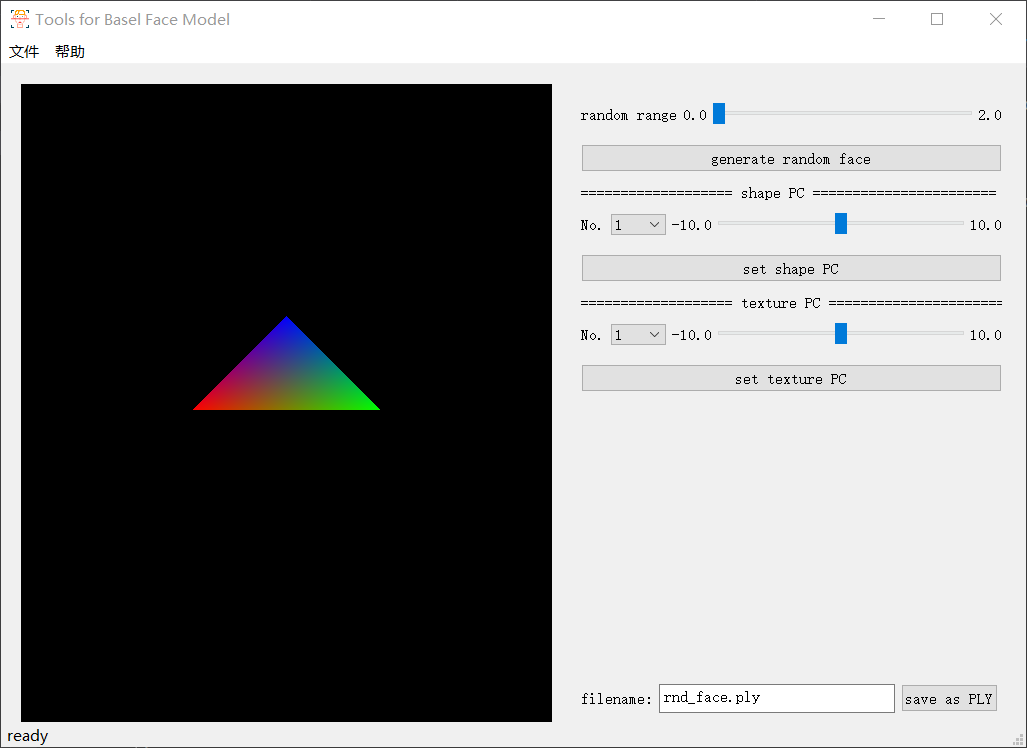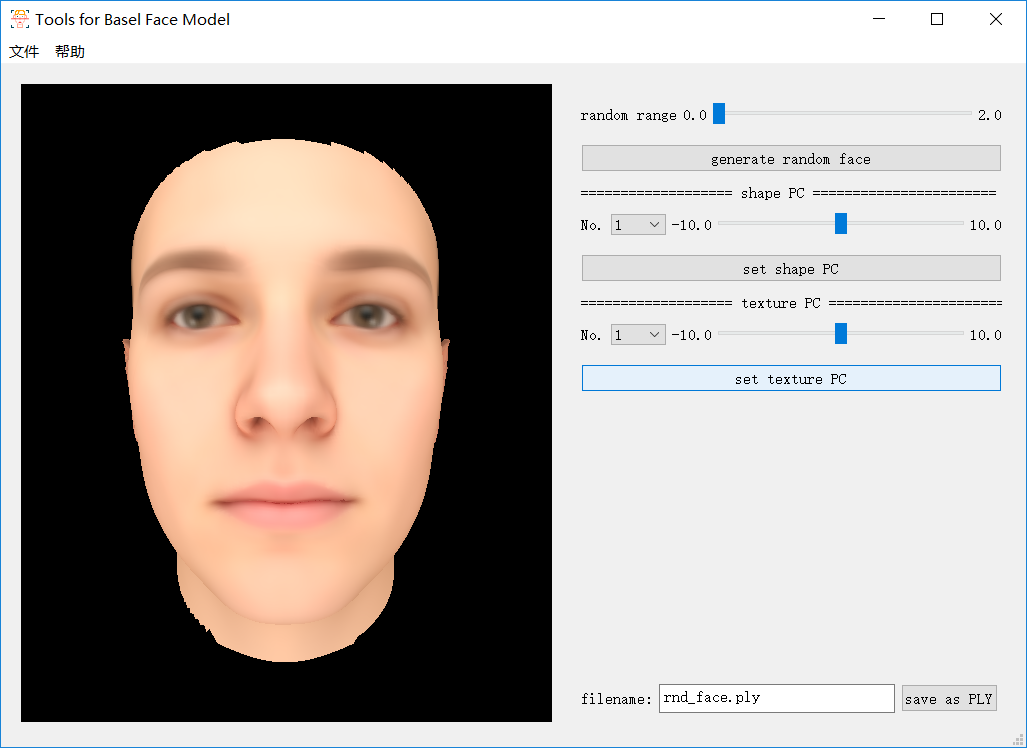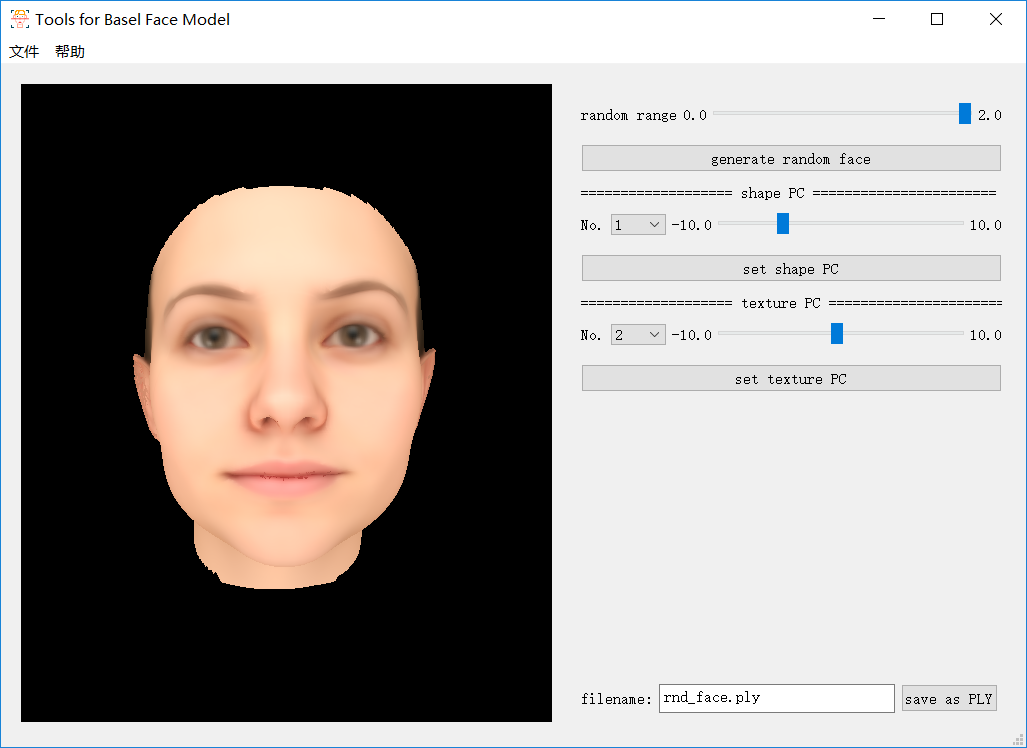### BFM模型介紹及可視化實現（C++）

• 2019 年 11 月 3 日
• 筆記

# BFM模型介紹及可視化實現（C++）

## BFM模型基本介紹

Basel Face Model是一個開源的人臉資料庫，其基本原理是3DMM，因此其便是在PCA的基礎上進行存儲的。

### 數據內容（以2009版本為例）

#### 文件內容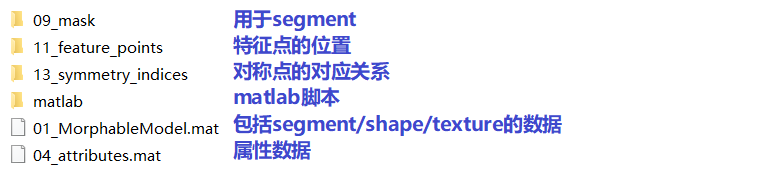#### 01_MorphableModel.mat（數據主體）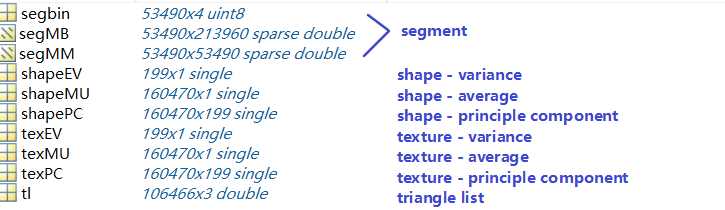BFM模型由53490個頂點構成，其shape/texture的數據長度為160470（53490*3），因為其排列方式如下：

``shape: x_1, y_1, z_1, x_2, y_2, z_2, ..., x_{53490}, y_{53490}, z_{53490}``
``texture: r_1, g_1, b_1, r_2, g_2, b_2, ..., r_{53490}, g_{53490}, b_{53490}``

#### .h5文件與.mat文件對應關係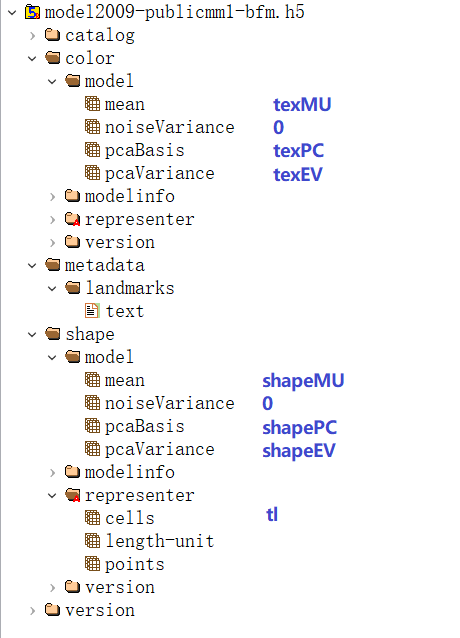[注] .h5文件中的tl數量與.mat數量不同，主成分方差的值也不同，且shape的值是.mat中shape值的0.001倍（見`/shape/representer/length-unit`）。

### 2009與2017版本區別

2009年版本數據集：

• 提供數據格式：mat（`01_MorphableModel.mat`）和h5（`model2009-publicmm1-bfm.h5`）；
• 提供一系列Matlab腳本，有生成隨機臉等功能；
• 提供多種特徵點（`PublicMM1/11_feature_points`）；
• 提供segment的mask（`PublicMM1/09_mask`）；
• 提供對稱點的對應關係（`PublicMM1/13_symmetry_indices`）；
• 提供屬性（`PublicMM1/04_attributes.mat`
• 不提供表情；

2017年版本數據集：

• 提供數據格式：h5（原版（`model2017-1_bfm_nomouth.h5`）和裁剪過的版本（`model2017-1_face12_nomouth.h5`））；
• 不提供Matlab腳本（本身也無mat格式數據）；
• 提供單種特徵點（`metadata/landmarks/text`）；
• 不提供segment、對稱點的對應關係和屬性；
• 提供表情（`expression`）；

### 基本原理

``obj = average + pc * (coeficient .* pcVariance)``

## C++實現BFM模型可視工具

### 數據讀取

#### 讀取.h5格式文件

.h5文件無法直接通過文本工具打開，需要下載專門的可視工具，此處我使用了HDFViewHDF5庫下載地址

[注] 在Windows的Visual Studio使用shared庫需要編譯過程定義`H5_BUILT_AS_DYNAMIC_LIB`。（若出現`LINK2001`錯誤可以添加這個來解決）
[注] static庫命名前面以`lib`開頭，例如`hdf5.lib`是shared庫，`libhdf5.lib`是static庫。

``float *shape_mu_raw = new float[N_VERTICE * 3];  H5File file(bfm_h5_path, H5F_ACC_RDONLY);  DataSet shape_mu_data = file.openDataSet("/shape/model/mean");  shape_mu_data.read(shape_mu_raw, PredType::NATIVE_FLOAT);  raw2vector(shape_mu, shape_mu_raw);   // 自行將數組轉換成想要存放的格式  shape_mu_data.close();  file.close();``

shape平均值讀取後需要再乘以1000才等同於.mat格式的讀取。

[注] 因為缺少pdb文件，HDF5中的程式碼如果報錯可能無法進行調試，需要逐行進行錯誤的排除，常見錯誤就是類型不匹配或者長度不匹配。

#### 其他讀取方式

``function mat2binary(filename, mat, type)      fid=fopen(filename, 'wb');      matrix = mat;                             [m,n]=size(matrix);       for i=1:1:m         for j=1:1:n              fwrite(fid, matrix(i,j), type);         end      end      fclose(fid);  end``

### OpenGL進行顯示

``double sint = sin(theta), cost = cos(theta);  for (auto t = tl.begin(); t != tl.end(); t++) {         glBegin(GL_TRIANGLES);         vec3 tmp = *t;         glColor3f(tex[tmp.x].x / 255.0, tex[tmp.x].y / 255.0, tex[tmp.x].z / 255.0);         glVertex3f(shape[tmp.x].x * scale * cost - shape[tmp.x].z * scale * sint, shape[tmp.x].y * scale, shape[tmp.x].x * scale * sint + shape[tmp.x].z * scale * cost);         glColor3f(tex[tmp.y].x / 255.0, tex[tmp.y].y / 255.0, tex[tmp.y].z / 255.0);         glVertex3f(shape[tmp.y].x * scale * cost - shape[tmp.y].z * scale * sint, shape[tmp.y].y * scale, shape[tmp.y].x * scale * sint + shape[tmp.y].z * scale * cost);         glColor3f(tex[tmp.z].x / 255.0, tex[tmp.z].y / 255.0, tex[tmp.z].z / 255.0);         glVertex3f(shape[tmp.z].x * scale * cost - shape[tmp.z].z * scale * sint, shape[tmp.z].y * scale, shape[tmp.z].x * scale * sint + shape[tmp.z].z * scale * cost);         glEnd();  }``

``void OpenGLWidget::resizeGL(int width, int height) {          glViewport(0, 0, width, height);          glMatrixMode(GL_PROJECTION);          glLoadIdentity();          gluPerspective(60.0, (GLfloat)width / (GLfloat)height, 1.0, 600000.0);          glMatrixMode(GL_MODELVIEW);          glLoadIdentity();          gluLookAt(0, 0, 300000.0, 0.0, 0.0, 0.0, 0.0, 1.0, 0.0);  }``

## 結果展示